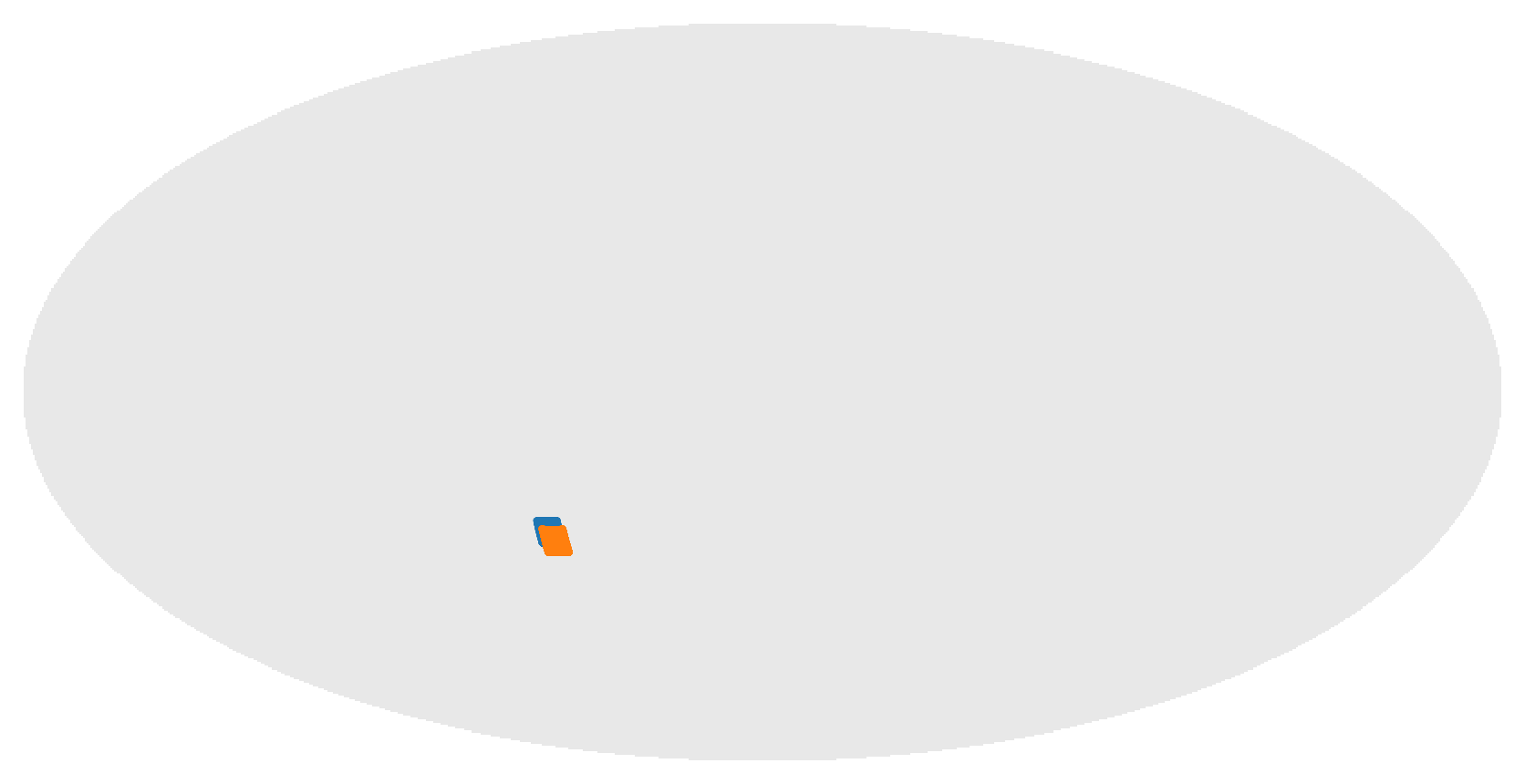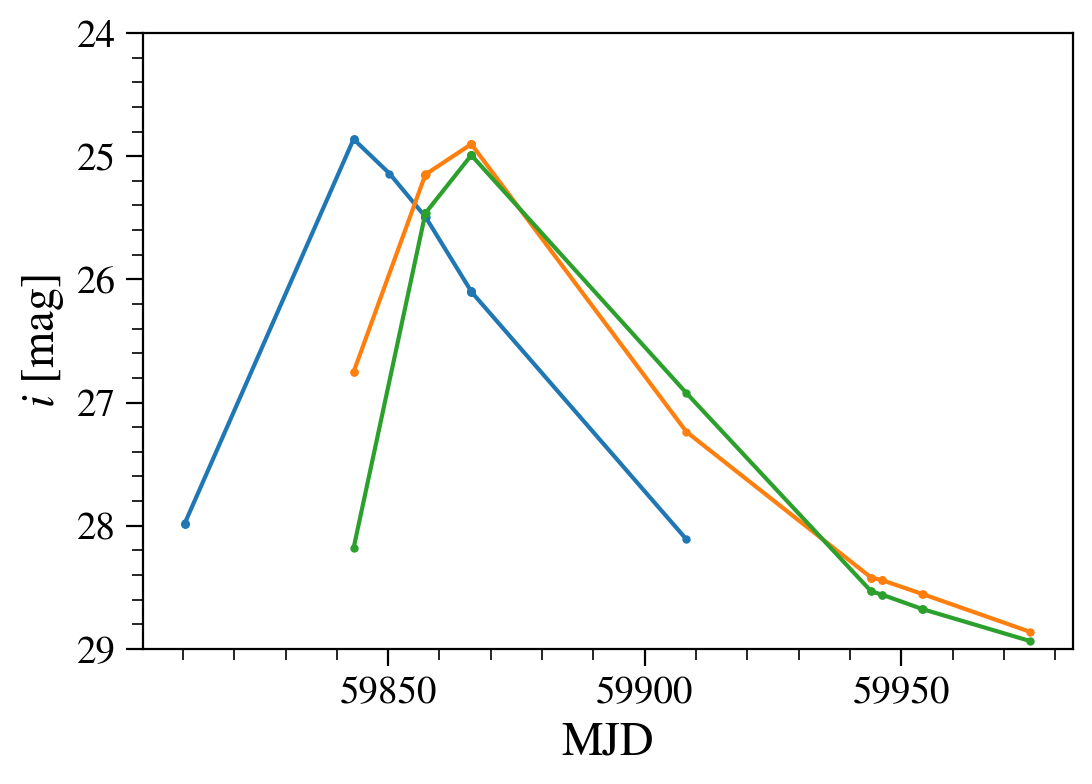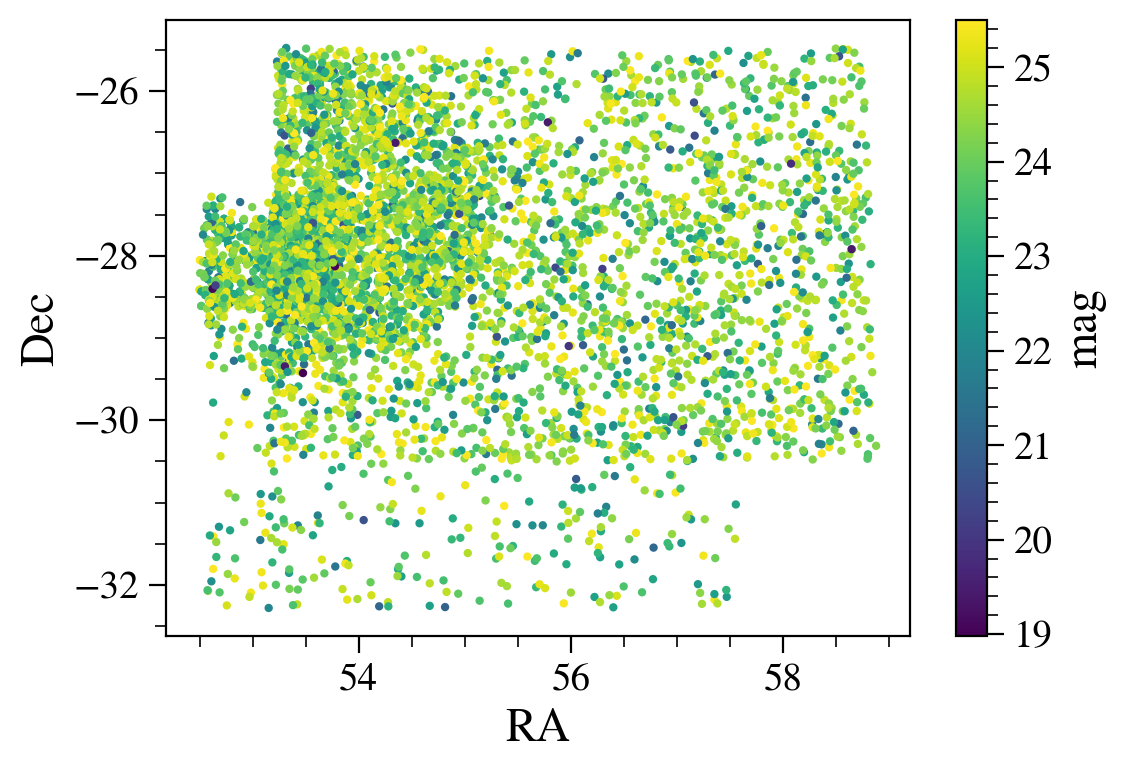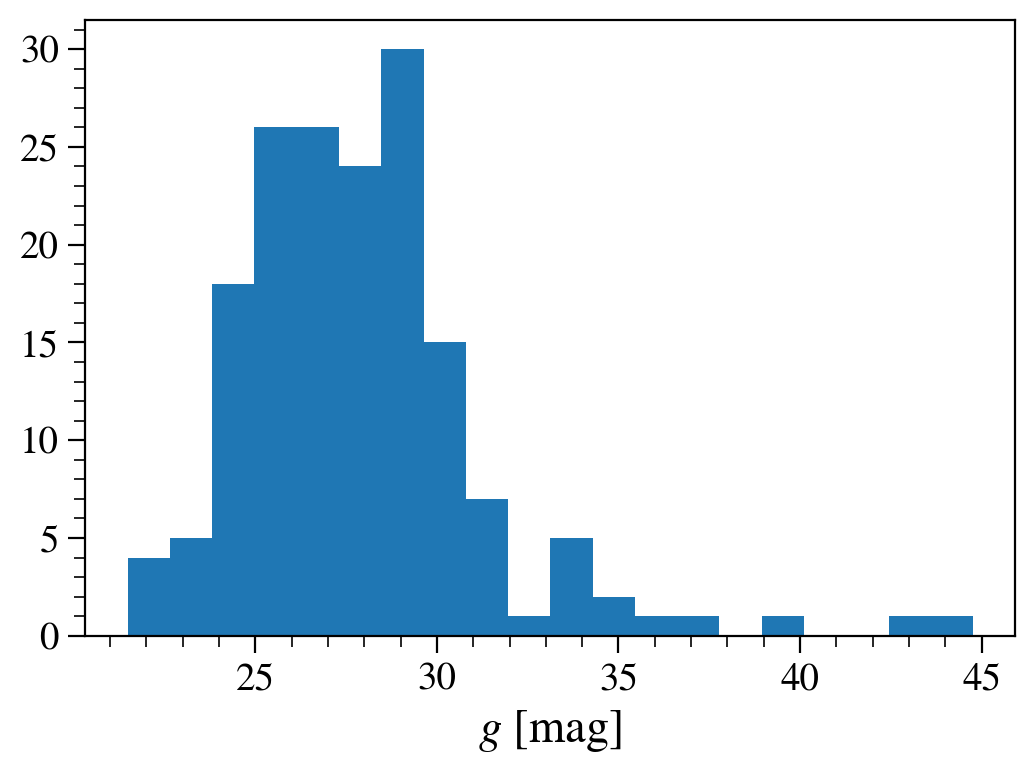# Accessing variable and transient objects in the truth catalog¶

Notebook owner: Yao-Yuan Mao @yymao.

Last Run: 2019-02-23

In addition to transients being included in images, information on the magnitudes of the transients are included in truth catalogs (input to the image simulation), which can eventually be compared to e.g., the fluxes determined from a difference image. This tutorial shows how to access the truth tables for these transients, and how to search for transients based on properties like magnitude or RA, Dec position.

Learning Objectives: Use GCR to load summary table and light curves of variable and transient objects in the truth catalogs

Notes:

In :
# import packages and methods that will be used in this notebook

import healpy as hp
import numpy as np
import GCRCatalogs
import matplotlib
%matplotlib inline
import matplotlib.pyplot as plt


## Summary catalog¶

First, we'll load the summary catalog (sc). The summary catalog is just a simple table that contains the coordinates, types, and id of the objects.

The filters and native_filters options work just like how they work for the static object catalog (see the truth_gcr_intro tutorial notebook).

In :
sc = GCRCatalogs.load_catalog('dc2_truth_run1.2_variable_summary')
sc.list_all_quantities(include_native=True)

Out:
['sprinkled', 'uniqueId', 'sn', 'agn', 'redshift', 'galaxy_id', 'ra', 'dec']

Let's now plot the locations of supernova and AGNs on the sky

In :
hp.mollview(np.ones(hp.nside2npix(2)), title='', cmap='Greys', min=0.9, max=1.5, cbar=None) # to get an empty sky map

data = sc.get_quantities(['ra', 'dec'], native_filters=['sn == 1'])
hp.projscatter(data['ra'], data['dec'], lonlat=True, s=1)

data = sc.get_quantities(['ra', 'dec'], native_filters=['agn == 1'])
hp.projscatter(data['ra'], data['dec'], lonlat=True, s=1)

/global/common/software/lsst/common/miniconda/py3.7-4.7.12.1-v2/envs/desc/lib/python3.7/site-packages/GCRCatalogs/dc2_truth.py:72: UserWarning: For this particular truth catalog, native_filters is no longer supported.
Please use filters instead. For now this code will include your native_filters in filters.
(Note that native_filters still works for other GCR catalogs.)
warnings.warn('For this particular truth catalog, native_filters is no longer supported.\n'

Out:
<matplotlib.collections.PathCollection at 0x2aab56858e90>## light curve catalog¶

The way to access light curve catalog (lc) is a bit different.

The data returned by the reader are the light curve tables, which have 5 columns: uniqueId, obshistid, mjd, filter, mag; and the filters option would work on any of these columns.

However, the native_filters option works differently. They would be imposed on the columns of the "summary catalog" we mentioned above, so that one can for example select SNs from a certain RA ranges.

Another difference is that, when return_iterator is turned on, the reader will iterator over the objects (i.e., returns one light curve table per objects).

It's probably easier to illustrate this by an example.

In :
lc = GCRCatalogs.load_catalog('dc2_truth_run1.2_variable_lightcurve')
lc.list_all_quantities(include_native=True)

Out:
['filter', 'uniqueId', 'obshistid', 'mjd', 'mag']

Let's plot the i-band light curves for 3 supernovae.

Note that here filter can be an integer of 0 to 5, and they correspond to ugrizy filters respectively.

In :
n_plotted = 0
for q in lc.get_quantities(['mjd', 'mag'], filters=['filter == 3'], native_filters=['sn == 1'], return_iterator=True):
if len(q['mjd']) >= 20:
plt.plot(q['mjd'], q['mag'], '.-')
n_plotted += 1
if n_plotted >= 3:
break
plt.ylim(29, 24)
plt.xlabel('MJD')
plt.ylabel('$i$ [mag]')

Out:
Text(0, 0.5, '$i$ [mag]')### Example 1: getting RA and Dec of all SNe whose brightest magnitude is brighter than a certain value¶

In this example, you will need to first iterate over the objects in the light curve table. You can do that by setting return_iterator=True. For each object, you then find the brightest r-band magnitude in the light curve, and see if it satisfies your criteria. If so, then you record the object ID (uniqueId), which you then use to get RA, Dec from summery table later.

In :
lc_data = []
for q in lc.get_quantities(['uniqueId', 'mag'], filters=['filter == 2'], native_filters=['sn == 1'], return_iterator=True):
if len(q['mag']) and q['mag'].min() < 25.5:
lc_data.append((q['uniqueId'], q['mag'].min()))
lc_data = np.array(lc_data, dtype=np.dtype([('uniqueId', int), ('mag_brightest', float)]))

In :
data = sc.get_quantities(['uniqueId', 'ra', 'dec'], filters=[(lambda x: np.in1d(x, lc_data['uniqueId'], True), 'uniqueId')])

In :
# let's check if all the IDs actually match
# Food for thought: is this always true? What if the order is not preserved between the two tables?

(data['uniqueId'] == lc_data['uniqueId']).all()

Out:
False
In :
plt.scatter(data['ra'], data['dec'], c=lc_data['mag_brightest'], s=4);
plt.xlabel('RA');
plt.ylabel('Dec');
plt.colorbar(label='mag');

# Food for thought: there seem to be different densities in different areas. Why?### Example 2: a histogram of brighest mag for all SNe in a particular area¶

This is an "opposite" task of Example 1. Here, we want to plot a histogram of brighest mag for all SNe in a particular area.

When querying the light curve table, you can use native_filters to filter on the columns of the summary table. Hence, for this task, you only need to use the light curve table (surprise!)

In :
area_cut = ['ra > 58', 'dec > -30', 'dec < -28']
sn_cut = ['sn == 1']
all_cuts = area_cut + sn_cut

mags = []
for q in lc.get_quantities(['mag'], filters=['filter == 1'], native_filters=all_cuts, return_iterator=True):
if len(q['mag']):
mags.append(q['mag'].min())

mags = np.array(mags)

In :
plt.hist(mags, 20);
plt.xlabel('$g$ [mag]');